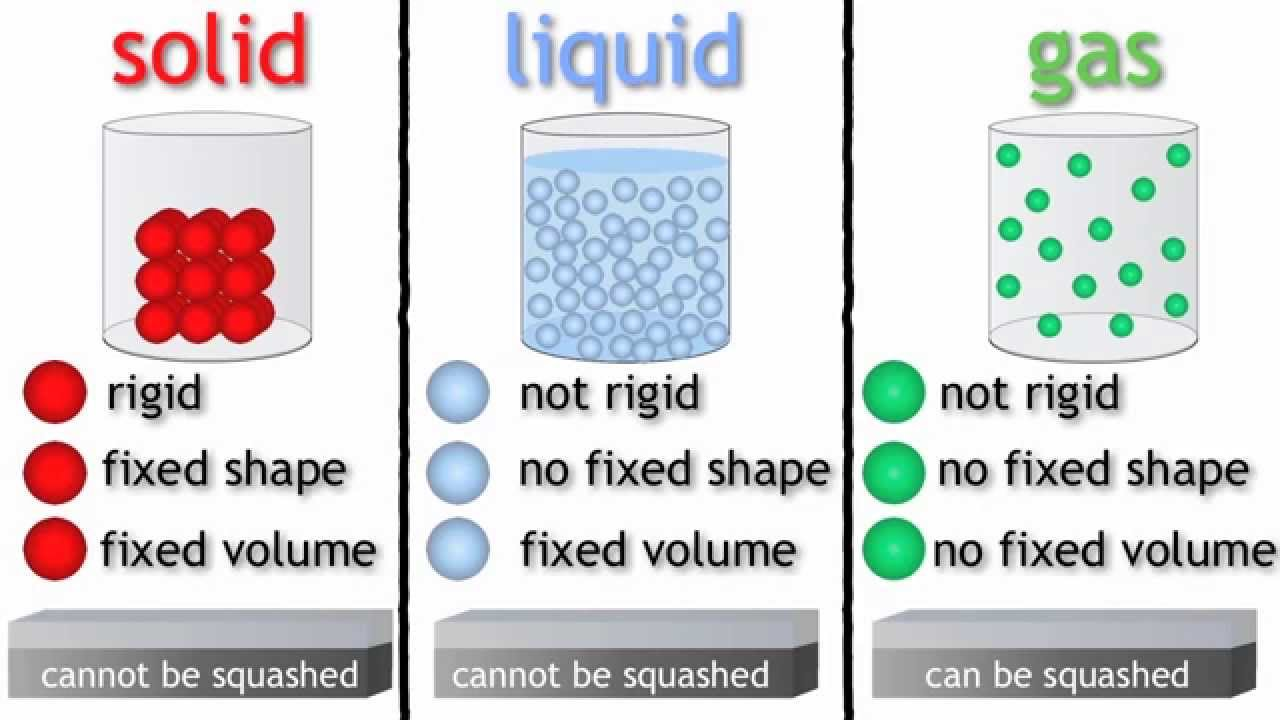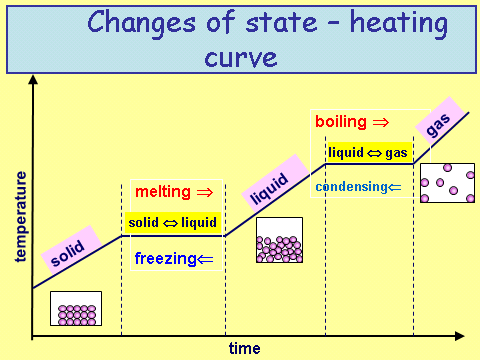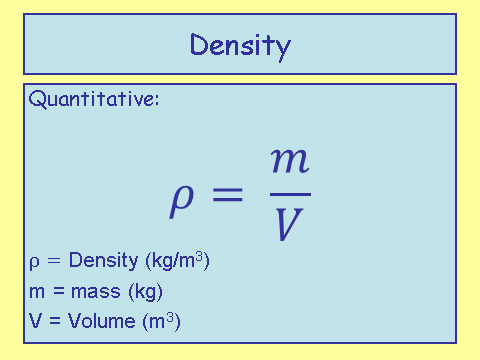# Density

## Solids Liquids and Gases

You must be able to draw a particle diagram (2D) of a solid, liquid and gasRules for drawing (to maximise marks)

Solids:

1. Must fill up the entire box
2. Must have a rigid pattern
3. Particles must be touching each other

Liquids:

1. Must fill up at least 2/3rds of the box
2. Must no have a rigid pattern - must be random
3. Particles must be touching each other

Gas:

1. Must fill up no more than 1/3rd of the box
2. Random order
3. Particles must not be touching each other

Out of the three; solids are the most dense with gases being the least (liquids are in-between).

## Heat Cooling Curve

The heating/cooling curve shows how a substances changes state as temperature changes with time.

Interestingly, the majority of this topic is contained within this graph.Notice when a substance changes state (i.e. melting, freezing etc) the temperature does not change. The reason for this is because the energy going into (or out of) the system is being used to break (or make) bonds between the molecules. Whilst this happens the temperature remains constant.

At this point it is important to give a new description to the term ‘temperature’, which is ‘a measure of how much the molecules are vibrating.’ The more they vibrate, the higher the temperature. In an exam you would not be asked for this description, but it does help you to imagine what is happening to the particles whilst they are changing state.

## Define Denisty

Density is a measure of how much mass is compacted into a given volume. The equation is one that you need to be able to recall and apply.Notice how the symbol for density is not the letter ‘p’, but is in fact a ‘⍴’. ⍴ is a greek letter called ‘rho’ and can be drawn as an italic ‘p’. If asked to write the equation, make sure you get the symbol right!! It might just be easier to write the density equation out in words.

Let’s look at some example calculations:

1. Calculate the density of an object which has a mass of 2kg and a volume of 15m3

2/15 = 0.13 kg/m3

1. Calculate the volume of an object which has a mass of 13000kg and a density of 25kg/ m3

13000/25 = 520 m3

1. Calculate the mass of an object which has a volume 70 m3 and a density of 0.2kg/ m3

70 x 0.2 = 14kg

Calculate the volume of an object which has a mass of 1400kg and a density of 100kg/m^3.
14
Calculate the mass of an object which has a volume 43.1 m^3 and a density of 26.6kg/m^3.